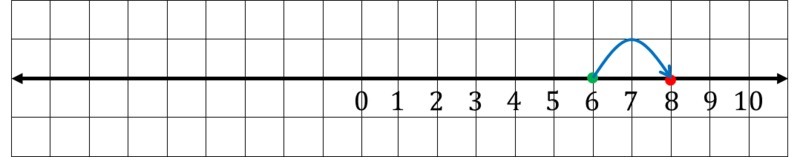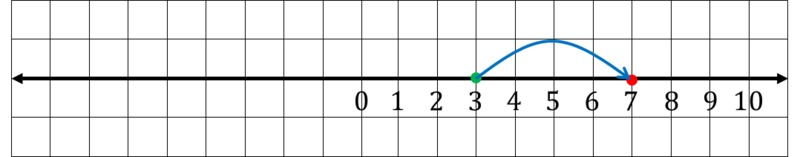# Extending the Number Line

Alignments to Content Standards: 6.NS.C.6

1. Draw a line on graph paper. Make a tick mark in the middle of the line and label it 0. Mark and label 1, 2, 3, ... 10. Since 6+2 is 2 units to the right of 6 on the number line, we can represent 6+2 like this:Describe the location of 3+4 on the number line in terms of 3 and 4. Draw a picture like the one above.

2. 6-2 is 2 units to the left of 6 on the number line, which we can represent like this:Describe the location of 3-4 on the number line in terms of 3 and 4. Draw a picture like the one above.

## IM Commentary

The purpose of this task is to understand that there are natural mathematical questions to ask for which there are no answers if we restrict ourselves to the positive numbers. The idea is to motivate the need for negative numbers and to see that there is a natural representation of them on the number line. In 7th grade, students will formally explore using a number line to add and subtract signed numbers.  This task does not aim to teach students to operate with integers on a number line, but simply to understand why we extend our number system beyond whole numbers. As students learn more about integer arithmetic and the number line, they will see, for part (b), that 3-4=-(-3+4)=-1.

This task could be used as a quick warm-up before a more formal introduction to negative numbers on the number line. In the solution, the negative numbers have not been marked on the number line: because this is an introductory task, students are not assumed to know how to label the negative numbers and the task serves as motivation for a discussion which can culminate in labeling the negative numbers.

## Solution

1. Since 3+4 is 4 units to the right of 3 on the number line, we can find and represent 3+4 like this:We can see from the number line that 3+4=7.

2. Since 3-4 is 4 units to the left of 3 on the number line, we can find and represent 3-4 like this:The difference 3-4 has a well determined place on the number line as shown in the picture. This place, however, is not marked and is not a whole number. The difference 3-4 is one unit to the left of 0.